# 5-Card Poker Hands

Brian Alspach

13 January 2000

### Abstract:

We determine the number of 5-card poker hands.

The types of 5-card poker hands are

• straight flush
• 4-of-a-kind
• full house
• flush
• straight
• 3-of-a-kind
• two pairs
• a pair
• high card

Most poker games are based on 5-card poker hands so the ranking of these hands is crucial. There can be some interesting situations arising when the game involves choosing 5 cards from 6 or more cards, but in this case we are counting 5-card hands based on holding only 5 cards. The total number of 5-card poker hands is.

A straight flush is completely determined once the smallest card in the straight flush is known. There are 40 cards eligible to be the smallest card in a straight flush. Hence, there are 40 straight flushes.

In forming a 4-of-a-kind hand, there are 13 choices for the rank of the quads, 1 choice for the 4 cards of the given rank, and 48 choices for the remaining card. This implies there are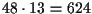4-of-a-kind hands.

There are 13 choices for the rank of the triple and 12 choices for the rank of the pair in a full house. There are 4 ways of choosing the triple of a given rank and 6 ways to choose the pair of the other rank. This produces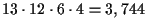full houses.

To count the number of flushes, we obtain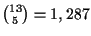choices for 5 cards in the same suit. Of these, 10 are straight flushes whose removal leaves 1,277 flushes of a given suit. Multiplying by 4 produces 5,108 flushes.

The ranks of the cards in a straight have the form x,x+1,x+2,x+3,x+4, where x can be any of 10 ranks. There are then 4 choices for each card of the given ranks. This yields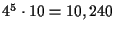total choices. However, this count includes the straight flushes. Removing the 40 straight flushes leaves us with 10,200 straights.

In forming a 3-of-a-kind hand, there are 13 choices for the rank of the triple, and there are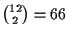choices for the ranks of the other 2 cards. There are 4 choices for the triple of the given rank and there are 4 choices for each of the cards of the remaining 2 ranks. Altogether, we have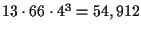3-of-a-kind hands.

Next we consider two pairs hands. There are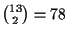choices for the two ranks of the pairs. There are 6 choices for each of the pairs, and there are 44 choices for the remaining card. This produces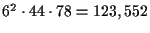hands of two pairs.

Now we count the number of hands with a pair. There are 13 choices for the rank of the pair, and 6 choices for a pair of the chosen rank. There are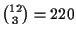choices for the ranks of the other 3 cards and 4 choices for each of these 3 cards. We have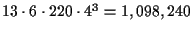hands with a pair.

We could determine the number of high card hands by removing the hands which have already been counted in one of the previous categories. Instead, let us count them independently and see if the numbers sum to 2,598,960 which will serve as a check on our arithmetic.

A high card hand has 5 distinct ranks, but does not allow ranks of the form x,x+1,x+2,x+3,x+4 as that would constitute a straight. Thus, there arepossible sets of ranks from which we remove the 10 sets of the form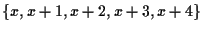. This leaves 1,277 sets of ranks. For a given set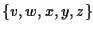of ranks, there are 4 choices for each card except we cannot choose all in the same suit. Hence, there are 1277(45-4) = 1,302,540 high card hands.

If we sum the preceding numbers, we obtain 2,598,960 and we can be confident the numbers are correct.

Here is a table summarizing the number of 5-card poker hands. The probability is the probability of having the hand dealt to you when dealt 5 cards.

 hand number Probability straight flush 40 .000015 4-of-a-kind 624 .00024 full house 3,744 .00144 flush 5,108 .0020 straight 10,200 .0039 3-of-a-kind 54,912 .0211 two pairs 123,552 .0475 pair 1,098,240 .4226 high card 1,302,540 .5012

Home | Publications | Preprints | MITACS | Poker Digest | Graph Theory Workshop | Poker Computations | Feedback
website by the Centre for Systems Science
last updated 12 January 2000